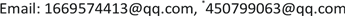﻿ 构造[[q2+1/53，q2+1/53 -2d+2，d]]q量子MDS码 Constructing [[q2+1/53，q2+1/53 -2d+2，d]]q Quantum MDS Code

Modern Physics
Vol. 09  No. 02 ( 2019 ), Article ID: 29498 , 6 pages
10.12677/MP.2019.92013

Constructing ${\left[\left[\frac{{q}^{2}+1}{53},\frac{{q}^{2}+1}{53}-2d+2,d\right]\right]}_{q}$ Quantum MDS Code

Meifang Zhao1, Pengfei Guo2*

1Mathematics Institute, South China University of Technology, Guangzhou Guangdong

2College of Computational Science, Zhongkai University of Agriculture and Engineering, Guangzhou GuangdongReceived: Mar. 5th, 2019; accepted: Mar. 20th, 2019; published: Mar. 28th, 2019ABSTRACT

Quantum MDS codes are an important class of quantum codes. In this paper, we construct new quantum MDS code ${\left[\left[\frac{{q}^{2}+1}{53},\frac{{q}^{2}+1}{53}-2d+2,d\right]\right]}_{q}$, the integer d belongs to $\left[2,18m+4\right]$ and is even if $q=106m+23$ and the integer d belongs to $\left[2,18m+4\right]$ and is even if $q=106m+83$.

Keywords:Conjugate Orthogonal, Quantum MDS Codes, Constacyclic Codes1华南理工大学数学学院，广东 广州

2仲恺农业工程学院计算科学学院，广东 广州Copyright © 2019 by authors and Hans Publishers Inc.1. 引言

$q$ 是一个素数的幂，码长为 $n$ 和码字个数为 $K$$q$ 元量子码 $Q$ 是指一个 ${q}^{n}$ 维希尔伯特空间 $H={\left(C{}^{q}\right)}^{\otimes n}={C}^{q}\otimes \cdots \otimes {C}^{q}$ 的一个 $k={\mathrm{log}}_{q}K$ 维子空间。用 ${\left[\left[n,k,d\right]\right]}_{q}$ 表示码长为 $n$ 最小距离为 $d$$q$ 元量子码，则此码可以检查 $\le d-1$ 错也可以纠正 $\le ⌊\frac{d-1}{2}⌋$ 位错  。特别地，对于量子码 ${\left[\left[n,k,d\right]\right]}_{q}$ 均须达到满足量子Singleton边界： $n\ge k+2d-2$。当量子码达到边界： $n=k+2d-2$，称为量子极大可分码(MDS码)。近些年构造良好性能的量子纠错码成为热门研究。同样利用Hermitian Construction理论，本文构造了一种新的MDS码。

2. 基本概念与预备知识

$x=\left({x}_{1},{x}_{2},\cdots ,{x}_{n}\right),y=\left({y}_{1},{y}_{2},\cdots ,{y}_{n}\right)\in {F}_{{q}^{2}}^{n}$，则 $x$$y$ 的Hermitian内积为 $\left(x,y\right)={\sum }_{i=1}^{n}{x}_{i}{\stackrel{¯}{y}}_{i}$，其中 ${\stackrel{¯}{y}}_{i}={y}_{i}^{q}$。如果 $\left(x,y\right)=0$，则称 $x$$y$ 正交。

$\eta =1$ 时， $C$ 为循环码；当 $\eta =-1$ 时， $C$ 为负循环码。

$\Omega =\left\{1+ir|0\le i\le n-1\right\}$ 对于 $\forall j\in \Omega$，集合 ${C}_{j}$ 表示包含 $j$$rn$${q}^{2}-$ 分圆陪集，即 ${C}_{j}=\left\{j,j{q}^{2},\cdots ,j{q}^{2\left(k-1\right)}\right\}\left(\mathrm{mod}rn\right)$$k$ 是满足 $j\equiv j{q}^{2k}\left(\mathrm{mod}rn\right)$ 的最小正整数。 $T=\left\{j\in \Omega |g\left({\omega }^{j}\right)=0\right\}$ 称为 $\eta -$ 常循环码 $C$ 的定义集。可以看到定义集 $T$ 是一些模 $rn$${q}^{2}-$ 分圆陪集的并而且 $\mathrm{dim}\left(C\right)=n-|T|$

$C$ 的生成式为 $g\left(x\right)={\prod }_{j\in T}\left(x-{\omega }^{j}\right)$，则 ${C}^{{\perp }_{H}}$ 的生成式为 ${g}^{{\perp }_{H}}\left(x\right)={\prod }_{j\in \Omega \begin{array}{c}\T\end{array}}\left(x-{\omega }^{-qj}\right)$。那么 ${C}^{{\perp }_{H}}\subseteq C$ 当且仅当 $g\left(x\right)|{g}^{{\perp }_{H}}\left(x\right)$。因此，我们有如下引理：

1) ${C}_{s}$ 是对称陪集当且仅当存在 $t\le ⌊\frac{m}{2}⌋$ 使得 $x\equiv x{q}^{2t+1}\left(\mathrm{mod}rn\right)$

2) 如果 ${C}_{y}\ne {C}_{z}$$\left({C}_{y},{C}_{z}\right)$ 形成一对不对称陪集当且仅当存在 $t\le ⌊\frac{m}{2}⌋$ 使得 $y\equiv y{q}^{2t+1}\left(\mathrm{mod}rn\right)$ 或者 $z\equiv z{q}^{2t+1}\left(\mathrm{mod}rn\right)$

1) $T\cap {T}^{-q}=\begin{array}{c}\varnothing \end{array}$，其中 ${T}^{-q}=\left\{-qs\mathrm{mod}rn|s\in T\right\}$

2) 如果 $i,j,k\in T$，那么 ${C}_{i}$ 不是偏斜非对称的和 $\left({C}_{j},{C}_{k}\right)$ 不是成对非对称陪集。

3. 量子MDS码构造

1) ${C}_{s}=\left\{s\right\}$${C}_{s+\frac{\left(q+1\right)n}{2}}=\left\{s+\frac{\left(q+1\right)n}{2}\right\}$

2) ${C}_{s-\left(q+1\right)j}=\left\{s-\left(q+1\right)j,s+\left(q+1\right)j\right\}$$1\le j\le \frac{n}{2}-1$

1) 如果 $q=106m+23$$0\le \delta \le 9m+1$，则 ${C}^{{\perp }_{H}}\subseteq C$

2) 如果 $q=106m+83$$0\le \delta \le 9m+6$，则 ${C}^{{\perp }_{H}}\subseteq C$

$s-\left(q+1\right)k\equiv -{q}^{\epsilon }\left(s-\left(q+1\right)l\right)\left(\mathrm{mod}rn\right),\epsilon =1,3$

1) 如果 $\epsilon =1$，那么

$s-\left(q+1\right)k\equiv -q\left(s-\left(q+1\right)l\right)\left(\mathrm{mod}\left(q+1\right)n\right)$

$s\equiv k+ql\left(\mathrm{mod}rn\right)$

${q}^{2}+1=106lq+106k\left(\mathrm{mod}2\left(q+1\right)\right)$

$106k=qt+h\left(0\le h\begin{array}{c}，则 $t=0,1,2,3,4,5,6,7,8$

① 若 $\left(106l+t\right)q+h={q}^{2}+1⇒h=1$，那么

$\left(106l+t\right)=q⇒t=23\left(\mathrm{mod}106\right)⇒t=23$ 矛盾。

② 若 $\left(106l+t\right)q+h=3{q}^{2}+3⇒h=3$，那么

$\left(106l+t\right)=3q⇒t=69\left(\mathrm{mod}106\right)⇒t=69$ 矛盾。

③ 若 $\left(106l+t\right)q+h=5{q}^{2}+5⇒h=5$，那么

$\left(106l+t\right)=5q⇒t=105\left(\mathrm{mod}106\right)⇒t=9$ 矛盾。

④ 若 $\left(106l+t\right)q+h=7{q}^{2}+7⇒h=7$，那么

$\left(106l+t\right)=7q⇒t=161\left(\mathrm{mod}106\right)⇒t=55$ 矛盾。

1) 如果 $\epsilon =3$，那么

$s-\left(q+1\right)k\equiv -{q}^{3}\left(s-\left(q+1\right)l\right)\left(\mathrm{mod}\left(q+1\right)n\right)$

$106lq\equiv 106k\left(\mathrm{mod}{q}^{2}+1\right)$

$106lq\equiv qt+h\left(\mathrm{mod}{q}^{2}+1\right)$

① 如果 $p=0$ 那么 $106lq=106k$。不可能成立。

② 如果 $p\ne 0$ 那么 $106lq=qt+h+p\left({q}^{2}+1\right)⇒h+p=\left(106l-t-pq\right)q⇒h\equiv -p\left(\mathrm{mod}q\right)⇒h=q-p$

$1\equiv 106l-t-p\left(106m+23\right)\left(\mathrm{mod}106\right)$

$1\equiv -t-23p\left(\mathrm{mod}106\right)$

$q=106m+23$，则 $n=\frac{{q}^{2}+1}{53}=212{m}^{2}+92m+10$$s=\frac{{q}^{2}+1}{2}=5618{m}^{2}+2438m+265$

$x,y\in Z=\left\{s+\left(q+1\right)j|0\le j\le 9m+1\right\}$。我们需要证明 $x+yq\ne 0\left(\mathrm{mod}rn\right)$ 成立。

$Z={\cup }_{i=1}^{5}{I}_{i}$${I}_{1}=\left[s,s+\left(q+1\right)m\right]$${I}_{2}=\left[s+\left(q+1\right)\left(m+1\right),s+\left(q+1\right)3m\right]$

${I}_{3}=\left[s+\left(q+1\right)\left(3m+1\right),s+\left(q+1\right)\left(5m+1\right)\right]$${I}_{4}=\left[s+\left(q+1\right)\left(3m+2\right),s+\left(q+1\right)\left(7m+1\right)\right]$

${I}_{5}=\left[s+\left(q+1\right)\left(7m+2\right),s+\left(q+1\right)\left(9m+1\right)\right]$

$x\in {I}_{1}$$26rn<26rn+\frac{\left({q}^{2}+1\right)\left(q+1\right)}{106}=s\left(q+1\right)\le x\left(q+1\right)\le \left[s+\left(q+1\right)m\right]\left(q+1\right)<27rn$

$x\in {I}_{2}$$27rn<\left[s+\left(q+1\right)\left(m+1\right)\right]\left(q+1\right)\le x\left(q+1\right)\le \left[s+\left(q+1\right)3m\right]\left(q+1\right)<28rn$

$x\in {I}_{3}$$28rn<\left[s+\left(q+1\right)\left(3m+1\right)\right]\left(q+1\right)\le x\left(q+1\right)\le \left[s+\left(q+1\right)\left(5m+1\right)\right]\left(q+1\right)<29rn$

$x\in {I}_{4}$$29rn<\left[s+\left(q+1\right)\left(5m+2\right)\right]\left(q+1\right)\le x\left(q+1\right)\le \left[s+\left(q+1\right)\left(7m+1\right)\right]\left(q+1\right)<30rn$

$x\in {I}_{5}$$30rn<\left[s+\left(q+1\right)\left(7m+2\right)\right]\left(q+1\right)\le x\left(q+1\right)\le \left[s+\left(q+1\right)\left(9m+1\right)\right]\left(q+1\right)<31rn$

$x,y\in {I}_{1}$ 时， $26rn

$x,y\in {I}_{2}$ 时， $27rn

$x,y\in {I}_{3}$ 时， $28rn

$x,y\in {I}_{4}$ 时， $29rn

$x,y\in {I}_{5}$ 时， $30rn

$x\in {I}_{1}\cup {I}_{2},y\in {I}_{1}$ 时， $26rn

$x\in {I}_{1}\cup {I}_{2}\cup {I}_{3},y\in {I}_{1}$ 时， $26rn

$x\in {I}_{1}\cup {I}_{2}\cup {I}_{3}\cup {I}_{4},y\in {I}_{1}$ 时， $26rn

$x\in {I}_{1}\cup {I}_{2}\cup {I}_{3}\cup {I}_{4}\cup {I}_{5},y\in {I}_{1}$ 时， $26rn

$x\in {I}_{2}\cup {I}_{3},y\in {I}_{2}$ 时， $27rn

$x\in {I}_{2}\cup {I}_{3}\cup {I}_{4},y\in {I}_{2}$ 时， $26rn

$x\in {I}_{2}\cup {I}_{3}\cup {I}_{4}\cup {I}_{5},y\in {I}_{2}$ 时， $26rn

$x\in {I}_{3}\cup {I}_{4},y\in {I}_{3}$ 时， $28rn

$x\in {I}_{3}\cup {I}_{4}\cup {I}_{5},y\in {I}_{3}$ 时， $28rn

$x\in {I}_{4}\cup {I}_{5},y\in {I}_{4}$ 时， $29rn。因此不存在偏斜非对称分圆陪集成对出现。

Constructing [[q2+1/53，q2+1/53 -2d+2，d]]q Quantum MDS Code[J]. 现代物理, 2019, 09(02): 114-119. https://doi.org/10.12677/MP.2019.92013

1. 1. 钱建发, 马文平. 量子纠错码的一个统一构造方法[J]. 计算机科学, 2010, 37(3): 70-72.

2. 2. Kai, X.S., Zhu, S.X. and Li, P. (2014) Constcyclic Codes and Some New Quantum MDS Codes. IEEE Transactions on Information Theory, 60, 2080-2086.
https://doi.org/10.1109/TIT.2014.2308180

3. 3. Kai, X.S. and Zhu, S.X. (2013) New Quantum MDS Codes from Negacyclic Codes. IEEE Transactions on Information Theory, 59, 1193-1197.
https://doi.org/10.1109/TIT.2012.2220519

4. 4. Taneja, D., Gupta, M., Narula, R. and Bhullar, J. (2017) Construction of New Quantum MDS Codes Derived from Constacyclic Codes. Interna-tional Journal of Quantum Information,15, 1750008-1-175008-12.
https://doi.org/10.1142/S0219749917500083

5. 5. Chen, B.C., Ling, S. and Zhang, G.H. (2015) Application of Constacyclic Codes to Quantum MDS Codes. IEEE Transactions on Information Theory, 61, 1474-1484.
https://doi.org/10.1109/TIT.2015.2388576

6. 6. Shi, X.,Yue, Q. and Zhu, X. (2017) Construction of Some New Quantum MDS Codes. Finite Fields and Their Applications, 46, 347-362.
https://doi.org/10.1016/j.ffa.2017.04.002

7. 7. Jin, L.,Ling, S., Luo, L. and Xing, C. (2010) Application of Classical Hermitian Self-Orthogonal MDS Codes to Quantum MDS Codes. IEEE Transactions on Information Theory, 56, 4735-4740.
https://doi.org/10.1109/TIT.2010.2054174

8. 8. 冯克勤, 陈豪. 量子纠错码[M]. 北京: 科学出版社, 2010.

9. 9. Yang, Y. and Cai, W. (2015) On Self-Dual Constacyclic Codes over Finite Fields. Designs, Codes and Cryptography, 74, 355-364.
https://doi.org/10.1007/s10623-013-9865-9

10. NOTES

*通讯作者。